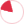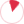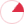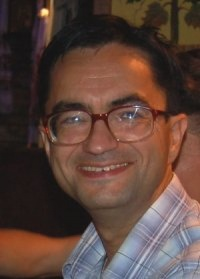## Schedule for Monday

 O.O. ShugailoWeek schedule# Vasyl O. Gorkavyy

Doctor of sciences in physics and mathematics, docent (associate professor)

## List of selected publications

Gorkavyy V. One integral inequality for closed curve in Euclidean space // C. R. Acad. Sci., Paris, Ser. I, v.321 (1995), №12, p.1587-1591,

Some integral inequalities for curvatures of closed curves in many-dimensional Euclidean space are proved.

Keywords: closed curve, curvature, Fary-Milnor inequality

Gorkavyy V. Reconstruction of a submanifold of Euclidean space from its Grassmannian image that degenerates into a line // Mathematical Notes, V.59 (1996), №5, p. 490-497,

Necessary and sufficient conditions for a curve in the Grassmann manifold to be a degenerate Gauss image of a manydimensional submanifold in Euclidean space are proved.

Keywords: Grassmann image, degenerate Grassmann image, asymptotic curve

Gorkavyy V. An analogue of the Grassmannian image for submanifolds of a sphere // Sbornik: Mathematics , V.187 (1996), №9, p.1283-1300,

Necessary and sufficient conditions for recovering a spherical submanifold from a given Gauss-Obata image are proved.

Gorkavyy V. Reconstruction of three-dimensional submanifolds of Euclidean space with large codimension from its Grassmannian image // Mathematical Notes, V.62 (1997), №5-6, p.581-585,

Necessary and sufficient analytical conditions for recovering a three-dimensional submanifold with large codimension in many-dimensional Euclidean space are derived.

Gorkavyy V. Theorem of reduction in the problem of reconstruction of sub-manifolds in Euclidean space from a given Grassmannian image // Matematicheskaya Fizika, Analiz, Geometria, V.4 (1997), №3, p.309-333,

It is shown that the reconstruction of an n-dimensional submanifolds with l-dimensional first normal space in (n+m)-dimensional Euclidean space from a given k-dimensional Gauss image may be reduced to the reconstruction of a k-dimensional submanifolds in (l+l)-dimensional Euclidean space from a given k-dimensional Gauss image.

Gorkaviy V. Deformations of surfaces F2 in E4 that preserve the Gauss image // Proceedings of the Conference "Geometry and Applications" dedicated to the seventieth birthday of V.A. Toponogov (Novosibirsk, Russia, March 13-17, 2000), P.34-57,

General and particular (conformal, isometric, equiareal) deformations of two-dimensional surfaces in four-dimensional Euclidean space that preserve the Gauss image are described. Rigidity theorems for particular classes of surfaces like minimal or isothermic ones are discussed.

Gorkavyy V. On pseudo-spherical congruencies in E4 // Matematicheskaya Fizika, Analiz, Geometria, V.10 (2003), №4, p.498-504,

An analogue of pseudo-spherical geodesic congruences in the four-dimensional Euclidean space is considered. A Baecklund theorem on the pseudo-sphericity of two-dimensional surfaces in E4 connected by pseudo-spherical geodesic congruencies is proved.

Gorkaviy V., Aminov Yu.A., Sviatovets A.V. On the reconstruction of particular closed surface in E4 from a given closed Grassmann image // Matematicheskaya Fizika, Analiz, Geometria, V.11 (2004), №1, p.3-24 ,

Theorems on the recovering of a particular closed rotationally symmetric surfaces in the four dimensional Euclidean space from a given Gauss image are proved.

Gorkavyy V. Bianchi congruencies for two-dimensional surfaces in Е4 // Sbornik: Mathematics, V.196 (2005), №9-10, p.1473-1493,

Two-dimensional pseudo-spherical surfaces in E4 that admit Bianci transformations are described.

Gorkavyy V. On pseudospherical surfaces in E4 with Grassmann image of prescribed type // Journal of mathematical physics, analysis, geometry, V.2 (2006), №2, p.138-148,

Following Yu.Aminov, there are three kinds of two-dimensional surfaces in the Grasmann manifold G(2,4), which are refewrred to as hyperbolic, parabolic and elliptic respectively. It is shown that there exist pseudo-spherical surfaces in E4, whose Gauss images are hyperbolic, parabolic or elliptic.

Gorkavyy V. On conformal transformations of surfaces in Minkowski space with preservation of the Gauss image // Russian Math. (Iz. VUZ), V.50 (2006), №7, p.11-21,

Two-dimensional surfaces in Minkowski space-time that admit conformal transformation preserving the Gauss image are described. Characterising theorems are proved for particular light-like surfaces, which were discovered earlier by K. Ilenko and may serve as light-like analogues of surfaces of null mean curvature.

Gorkavyy V. On minimal lightlike surfaces in Minkowski space-time // Differential Geometry and its Applications, V.26 (2008), №2, p.133-139,

Two-dimensional light-like surfaces in Minkowski space-time that admit isometric trasformations preserving the Gauss image are described.

Gorkavyy V. On pseudo-spherical congruencies in spaces of constant curvature // Annals on the National Academy of Sciences of Ukraine, (2008), №6, p.13-18,

Pseudo-spherical geodesic congruencies in spherical and hyperbolical spaces are considered. A Backlund type theorem on the pseudo-sphericity of two-dimensional surfaces connected by pseudo-spherical geodesic congruencies is proved.

Gorkavyy V.O. , Nevmerzhytska O.M. Ruled surfaces as pseudo-spherical congruencies // Journal of mathematical physics, analysis, geometry , V.5 (2009), №4, p.359-374,

We classify ruled surfaces in constant curvature spaces En, Sn, Hn and in Riemannian products SnxR1, HnxR1, whose transformations of shifts along rulings may generate pseudo-spherical congruencies.

Gorkavyy V.A., Milka A.D. Volume increasing linear bendings of right polyhedra // Proceedings of Institute of mathematics of NAS of Ukraine, V.6 (2009), №2, p.152-182,

We construct particular linear bending of an arbitrary right pyramid, which increase the volume of pyramid. Consequently, for every convex right polyhedron we construct an iterating linear bending, which increase the volume of polyhedron. This improves previous numerical results obtained by D.D. Bleecker. For instance, the achived relative volume increase is equal to 1.44+ for the tetrahedron, 1.24+... for the cube, 1.13589+ for the octahedron, 1.09723+ for the dodecahedron, 1.04272+ for the icosahedron. Linear bendings with more efficient volume growth are still desired.

Gorkavyy V.A., Milka A.D., Soboleva A.N. Volume increasing linear bendings of prisms // Рroc. Intern. Geom. Center dω, V.2 (2010), №1, p.7-26,

We construct and analyze particular iterating linear bendings, which increase the volume, for right prisms.

Gorkavyy V. On inflating closed mylar shells // Comptes Rendus. Mecanique, V.338 (2010), №12, p.656-662,

Following ideas of W. Paulsen, we discuss particular short deformations, which increase the volume, for a large class of closed surfaces of rotation.

Gorkavyy V., Nevmerzhytska O. Аn analogue of the Bianchi transformation for two-dimensional surfaces in the space S3 x R1 // Mathematical Notes, V.89 (2011), №6, p.833-845,

An analogue of the classical Bianchi transformation is constructed for surfaces in the Riemannian product space S3xR1.

Gorkavyy V.A. An Example of Bianchi Transformation in E4 // Journal of mathematical physics, analysis, geometry, V.8 (2012), p. 240–247,

We construct examples of two-dimensional pseudo-spherical surfaces, which admit Bianchi transformations, in the four-dimensional Euclidean space.

Gorkavyy V., Nevmerzhitska O. Pseudo-spherical submanifolds with degenerate Bianchi transformation // Results in mathematics, V. 60 (2011), №1, p.103-116,

We classify pseudo-spherical submanifolds, which admit a Bianchi transformation degenerated into a line, in spaces of constant curvature.

Gorkavyy V. A generalisation of Bianchi-Baecklund transformation of pseudo-spherical surfaces // Itogi nauki i techniki. Seria "Sovremennaya mathematika i ee prilozheniya. Tematicheskie obzory", V. 126 (2014), p. 191-218,

В обзоре приведены основные результаты по обобщению геометрического преобразования Бианки-Беклунда на случай псевдо-сферических поверхностей в многомерных пространствах постоянной кривизны En, Sn, Hn и в произведениях Sn x R1, Hn x R1.